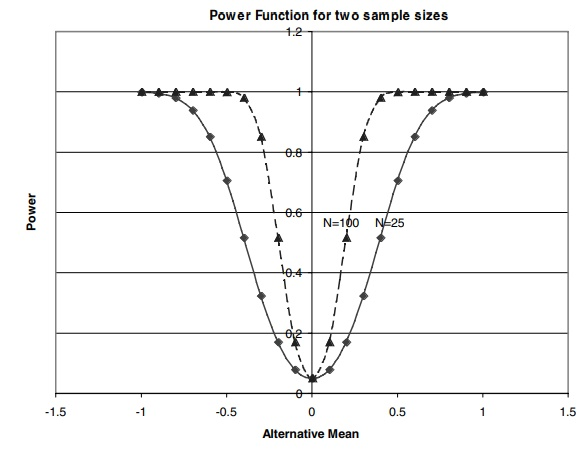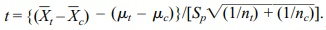# Two-Sample t Test (Independent Samples with a Common Variance)

| Home | | Advanced Mathematics |

## Chapter: Biostatistics for the Health Sciences: Tests of Hypotheses

Power function for a test that a normal population has mean zero versus a two-sided alternative

TWO-SAMPLE t TEST (INDEPENDENT SAMPLES WITH A COMMON VARIANCE)

Recall from Section 8.5 the use of the appropriate t statistic for a confidence interval under the following circumstances: the parent populations have normal distributions and common variance that is unknown. In this situation, we used the pooled variance estimate, s2p, calculated by the formula Sp2 = {St2(nt – 1) + Sc2(nc – 1)}/[nt + nc – 2]Figure 9.2. Power function for a test that a normal population has mean zero versus a two-sided alternative when the sample size n = 25, n = 100, and the significance level α = 0.05.

Suppose we want to evaluate whether the means of two independent samples selected from two parent populations are significantly different. We will use a t test with sp2 as the pooled variance estimate. The corresponding t statistic is t. The formula for t is obtained by replacing the common in the formula for the two sample Z test with the pooled estimate Sp. The resulting statistic has Student’s t distribution with nt + nc – 2 degrees of freedom. This sample t statistic is used for hypothesis testing. For a two-sided test the steps are as follows:

1. State the null hypothesis H0: μt = μc versus the alternative hypothesis H1: μt

≠ μc.

2. Choose a significance level α= α0 (often we take α0 = 0.05 or 0.01).

3. Determine the critical region, that is, the region of values of t in the upper and lower α/2 tails of the sampling distribution for Student’s t distribution with nt + nc – 2 degrees of freedom when μt = μc (i.e., the sampling distribution when the null hypothesis is true).

4. Compute the t statistic:for the given sample and sample sizes nt and nc, where Xt is the sample mean for the treatment group,c is the sample mean for the control group, and Sp is the pooled sample standard deviation.

5. Reject the null hypothesis if the test statistic t (computed in step 4) falls in the rejection region for this test; otherwise, do not reject the null hypothesis.

We will apply these steps to the pig blood loss data from Section 8.7, Table 8.1. Recall that Sp2 = {S2t(nt – 1) + Sc2(nc – 1)}/[nt + nc – 2] = {(717.12)2 9 + (1824.27)2 9}/18, since nt = nc = 10, St = 717.12, and Sc = 1824.27. So Sp2 = 2178241.61 and taking the square root we find Sp = 1475.89. As the degrees of freedom are nt + nc – 2 = 18, we find that the constant C from the table of the Student’s t distribution is 2.101. Applying steps 1–5 to the pig blood loss data for a two-tailed (two-sided) test, we have:

1. State the null hypothesis H0: μt = μc versus the alternative hypothesis H1: μt ≠ μc.

2. Choose a significance level α = α0 = 0.05.

3. Determine the critical region, that is, the region of values of t in the upper and lower 0.025 tails of the sampling distribution for Student’s t distribution with 18 degrees of freedom when μt/μc (i.e., the sampling distribution when the null hypothesis is true).

4. Compute the t statistic:We are given that the sample sizes are nt = 10 and nc = 10, respectively. Under the null hypothesis, μtμc = 0 andtXc = 1085.9–2187.4 = –1101.5 and sp, the pooled sample standard deviation, is 1475.89. Since {(1/nt) + (1/nc)]} = (2/20) = 0.1 = 0.316, t = –1101.5/(1475.89)0.316 = –2.362.

5. Now, since –2.362 < –C = –2.101, we reject H0.